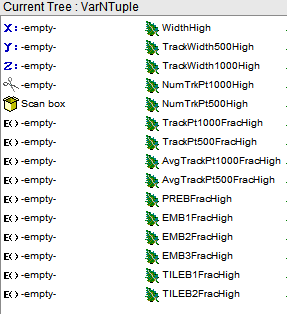# Accessing the data in ntuples

Hello,
I have seen some similar topics here but cannot find quite what I’m looking for.
I used EventLoop to create ntuples, following the tutorial for ATLAS at:
[ATLAS Analysis Software Tutorial | Creating and Filling Trees/NTuples]
Each variable is filled into a vector of floats which is then filled into the tree.
I want to work with the resulting ntuple, going through each variable and calculating simple things like mean and covariance.
The problem is that I cannot seem to extract the data from the ntuple. Here is what my Tree looks like:I have tried following instructions that involve

``````TFile *mainInput = new TFile("path/to/file", "READ");
TTree* mainTree = (TTree*)mainInput->Get("VarNTuple");

std::vector<float>* WidthHigh;

``````

But any attempts to Draw or scan or show after this are failing.
What do I need to do to access data from a Tree of vectors like this?
Thanks,
Alex

At least: `std::vector<float> *WidthHigh = 0;`

Here’s what you should do:

``````ROOT::EnableImplicitMT(); // use all cores
ROOT::RDataFrame rdf("VarNTuple", "path/to/file");
auto hist = rdf.Histo1D("EMB1FracHigh");
auto meanTrackPt500 = rdf.Mean("AvgTrackPt500FracHigh");
std::cout << "meanTrackPt500 = " << *meanTrackPt500 << '\n';
hist->Draw();
``````

This is an example showing a histogram and an average; you can do way more, see the documentation. This is way faster, and it’s way easier for you to evolve this into a full-blown analysis.